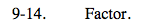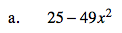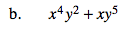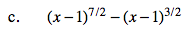### Home > CAAC > Chapter 16 > Lesson 16.9.1.1 > Problem9-14

9-14.This is the difference of two perfect squares.Factor out the greatest common factor. Then factor again since you have a binomial with two perfect cubes.

$xy^{2}(x^{3} + y^3)$

$xy^{2}(x + y)(x^{2} - xy + y^2)$$\text{Factor out }(x - 1)^{3/2}$

$\text{ from both sides.}$

$(x - 1)^{3/2}\left((x - 1)^{4/2} - 1\right)$

$(x - 1)^{3/2}\left((x - 1)^{2} - 1\right)$